Electrical Forces Coulomb's Law

Electrical Forces Coulomb’s Law

In the previous sections we learned that same charges repel each other and opposite charges attract each other. Experiments done on this subject shows that this force is depends on the distance between the charges and amount of charges.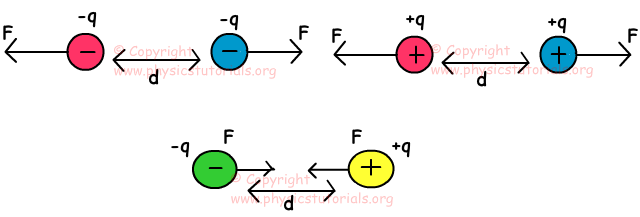If the distance between the charged object increase, then amount of attraction or repulsion decrease. On the contrary if the distance between them decreases then amount of force increases. Moreover, electrical forces are directly proportional to the amount of net charge. Coulomb made some experiments and find following equation of electrical forces.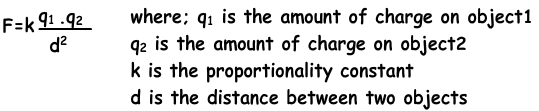· If the objects have same type of charge then the force is repulsive, if they have opposite charges then force is attractive.

· Repulsive or attractive electrical forces is equal in magnitude but opposite in direction, it does not depend on the magnitude

F12=-F21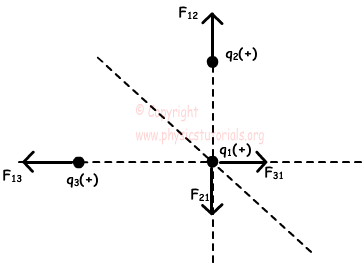Picture given above shows the forces acting on the charges. F21 is the force of repulsion of F2 on F1; F12 is the force of F1 on F2. As I said before;

F12=-F21

Example: If the q2 charge does not move, find the charge of q3 in terms of q?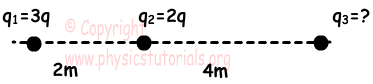Example: If the net force acting on q2 is F2 find the charge of q3 in terms of q?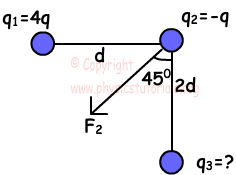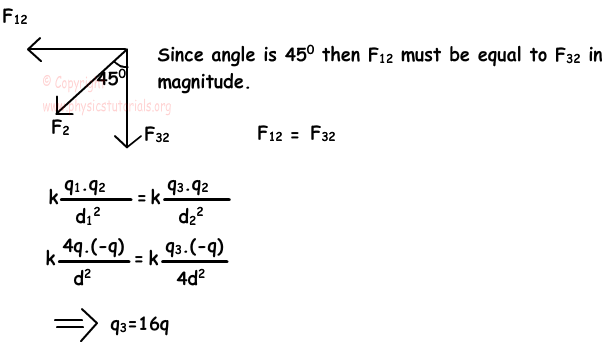Example: If the system given below is in equilibrium, find the q in terms of given quantities and tension in the rope in terms of mg.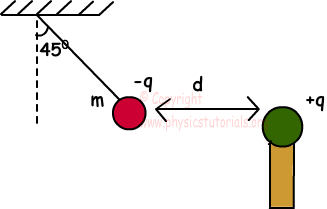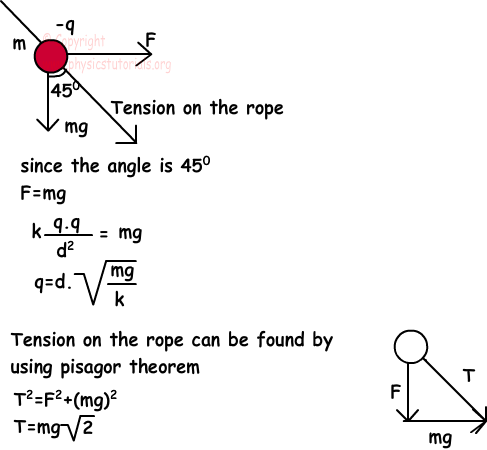Electrostatics Exams and Solutions

Author: